# Introduction

Wicherts (2016) argues that there are multiple problems with using Jensen’s method (method of correlated vectors) on item level data, such as has been done by te Nijenhuis et al (many papers) and Kirkegaard (2015). One of the problems involves the assumption that item-total Pearson correlations can be used as estimates of ‘g-loadings’ of items. If we assume for simplicity a simplified scale that consists entirely of common factor variance and error variance, then the Pearson correlation between the total score and the item depends on two values:

1. The item’s discriminateness.
2. The item’s pass rate.

The reason for the second is that because a single item is a dichotomous score, its variance depends on the pass rate (base rate). Consider a simple example where each item has a discriminateness. (item response theory) of 1.0, but different pass rates:

#generate data
set.seed(1)
d = data_frame(
total_score = rnorm(1e3),
item_1 = as.numeric(total_score > qnorm(.50)), #threshold at 50%
item_2 = as.numeric(total_score > qnorm(.60)), #at 60%
item_3 = as.numeric(total_score > qnorm(.70)), #at 70%
item_4 = as.numeric(total_score > qnorm(.80)), #at 80%
item_5 = as.numeric(total_score > qnorm(.90))  #at 90%
)

#observed Pearson correlations
cor(d)
##             total_score item_1 item_2 item_3 item_4 item_5
## total_score        1.00   0.80   0.79   0.76   0.70   0.60
## item_1             0.80   1.00   0.84   0.69   0.52   0.36
## item_2             0.79   0.84   1.00   0.81   0.62   0.43
## item_3             0.76   0.69   0.81   1.00   0.77   0.53
## item_4             0.70   0.52   0.62   0.77   1.00   0.69
## item_5             0.60   0.36   0.43   0.53   0.69   1.00
#variances
var(d)
##             total_score item_1 item_2 item_3 item_4 item_5
## total_score        1.07  0.412  0.400  0.361  0.292  0.193
## item_1             0.41  0.250  0.207  0.158  0.106  0.056
## item_2             0.40  0.207  0.240  0.183  0.123  0.065
## item_3             0.36  0.158  0.183  0.212  0.142  0.075
## item_4             0.29  0.106  0.123  0.142  0.163  0.086
## item_5             0.19  0.056  0.065  0.075  0.086  0.096
#density of normal durve at the same z-scores
dnorm(qnorm(seq(.5, .9, .1)))
##  0.40 0.39 0.35 0.28 0.18

Thus, we observe that the Pearson correlations fall in perfect accordance with the variance of the items, making this a special case of variance reduction (‘restriction of range’). Notice the relationship between the item variances and the densities of the normal curve at the same z values used to create the items. Given such a regularity, readers may wonder whether it is possible to estimate what the Pearson correlation would have been if the items were not dichotomized, and it is. The formula is given by Hunter and Schmidt (2004; rearranged for readability):

$r_{true} = r_{observed} \cdot (\frac{d(z)}{sqrt(P \cdot Q)})^{-1}$ where P is the proportion of cases above the threshold (pass rate), Q is the proportion below (so Q = 1-P), and d is the density of the normal curve at the z score used to dichotomize the distribution. For example, for the first item, these values are:

$r_{true} = .80 \cdot (\frac{.40}{sqrt(.5 \cdot .5)})^{-1} = .80 \cdot (\frac{.40}{.5})^{-1} = .80 \cdot .80^{-1} = 1$

and for the item with a pass rate of .10:

$r_{true} = .60 \cdot (\frac{.18}{sqrt(.1 \cdot .9)})^{-1} = .60 \cdot (\frac{.18}{.30})^{-1} = .60 \cdot .60^{-1} = 1$

This way of estimating what a correlations would have been is a special class of latent correlations called the biserial correlation (not to be confused with the point-biserial correlation).

# Jensen’s method applied to item pass rates: a simulated example

Before trying the corrected Jensen’s method, let’s first see the problem Wicherts is talking about. To do that we have to generate some data. We will use 100 items, although more commonly the 60 items of the Raven’s Standard Progressive Matrices have been used. Using more reduces indicator (psychometric) sampling error which we are not interested in here. As before, we assume a single factor structure (á la Spearman’s original model) with only two sources of variance: g variance and error variance. We also let the items vary randommly in their pass rates and their discriminateness.

#we generate the item data
set.seed(1)
d2_items = data_frame(
#id
id = 1:100,
#generate some item pass rates
pass_rate = pnorm(rnorm(100)),
#their z score equivalents  = item difficulty in IRT
item_difficulty = qnorm(pass_rate, lower.tail = F),
#item discriminatively
discrimination = runif(100, min = .1, max = 2)
)

#item col names
d2_item_names = "item_" + 1:nrow(d2_items)

#sample size
simul_n = 3e4

Having set up our item data, we generate 30000 cases from three groups with mean abilities of -.50, 0 and .50.

set.seed(1)
#generate groups
d2_data = data_frame(group = rep(LETTERS[1:3], length.out = simul_n))

#generate person latent ability levels
d2_data$latent_ability = NA d2_data[d2_data$group == "A", "latent_ability"] = rnorm(nrow(d2_data)/3, mean = -.5)
d2_data[d2_data$group == "B", "latent_ability"] = rnorm(nrow(d2_data)/3, mean = 0) d2_data[d2_data$group == "C", "latent_ability"] = rnorm(nrow(d2_data)/3, mean = .5)

#generate item scores
#for each item
set.seed(1)
for (item in 1:nrow(d2_items)) {
#u, from 2PN
x = d2_items$discrimination[item] * (d2_data$latent_ability - d2_items$item_difficulty[item]) #chance of getting item right for each person y = pnorm(x, lower.tail = F) #roll the dice and save output d2_data[["item_" + item]] = (runif(nrow(d2_data)) > y) } #total score (sum) d2_data$total = rowSums(d2_data[-c(1:2)])

#plot
ggplot(d2_data, aes(total)) +
geom_density(fill = "black", alpha = .3) +
xlab("Total score")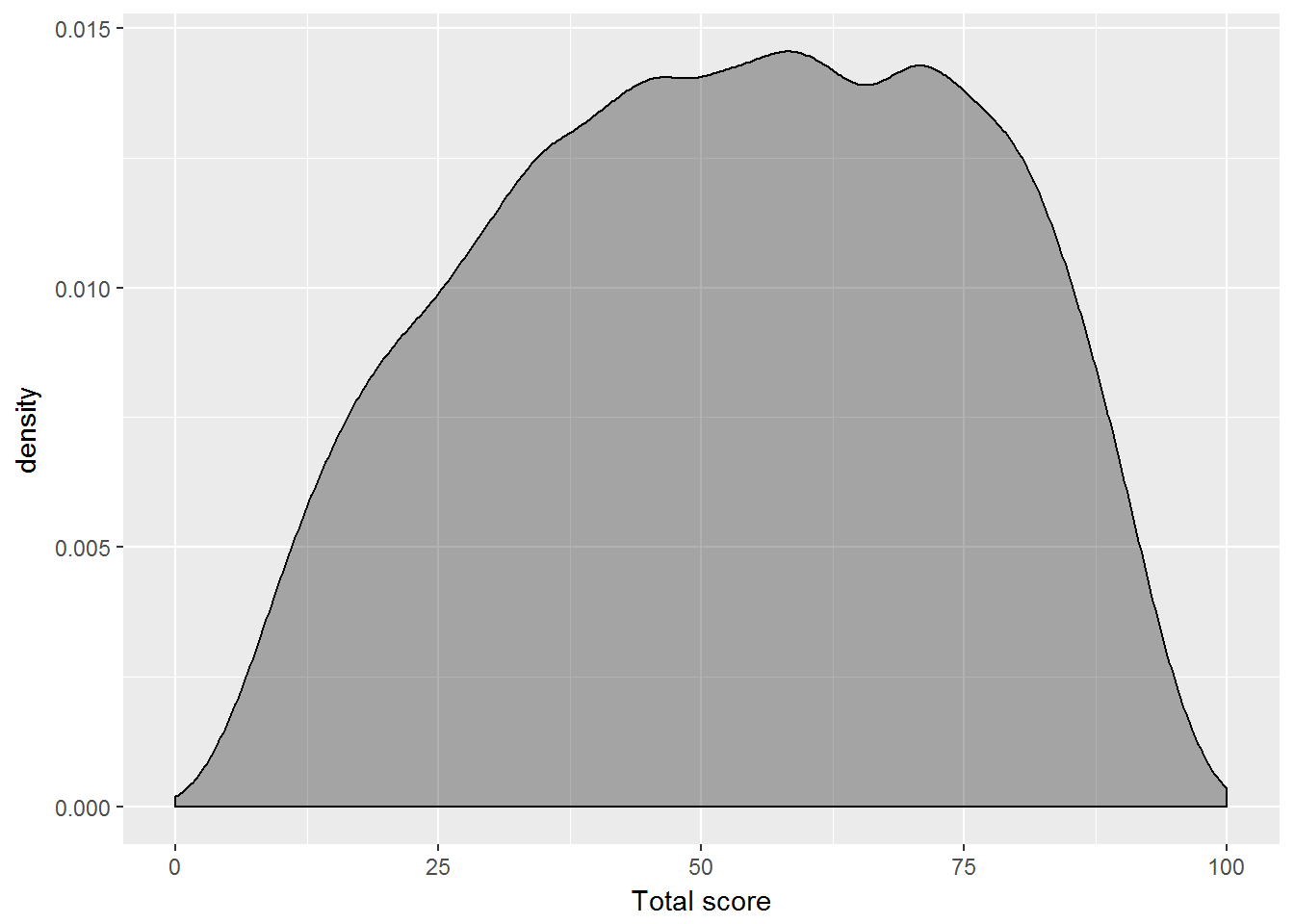ggplot(d2_data, aes(total, fill = group, color = group)) +
geom_density(alpha = .3) +
xlab("Total score")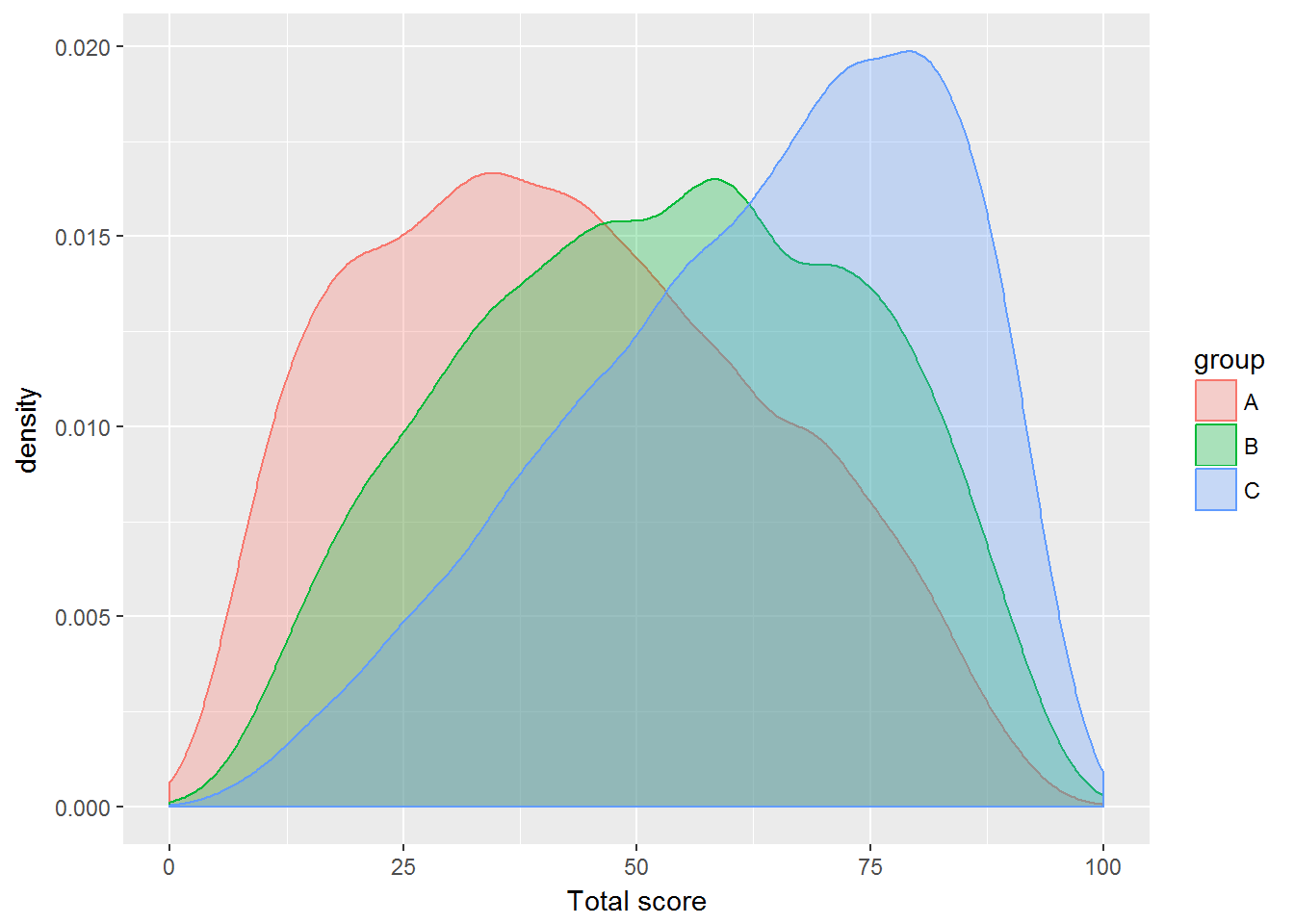#reorder
d2_data = df_reorder_columns(d2_data, c("group" = 1, "latent_ability" = 2, "total" = 3))

#empirical pass rates
d2_items$pass_rate_empirical = colMeans(d2_data[-(1:3)]) #inspect some Pearson correlations cor(d2_data[2:10]) ## latent_ability total item_1 item_2 item_3 item_4 item_5 ## latent_ability 1.00 0.97 0.43 0.39 0.57 0.39 0.34 ## total 0.97 1.00 0.44 0.40 0.57 0.40 0.36 ## item_1 0.43 0.44 1.00 0.17 0.26 0.17 0.14 ## item_2 0.39 0.40 0.17 1.00 0.22 0.15 0.14 ## item_3 0.57 0.57 0.26 0.22 1.00 0.20 0.19 ## item_4 0.39 0.40 0.17 0.15 0.20 1.00 0.13 ## item_5 0.34 0.36 0.14 0.14 0.19 0.13 1.00 ## item_6 0.57 0.57 0.27 0.22 0.38 0.20 0.19 ## item_7 0.61 0.63 0.26 0.24 0.33 0.27 0.22 ## item_6 item_7 ## latent_ability 0.57 0.61 ## total 0.57 0.63 ## item_1 0.27 0.26 ## item_2 0.22 0.24 ## item_3 0.38 0.33 ## item_4 0.20 0.27 ## item_5 0.19 0.22 ## item_6 1.00 0.33 ## item_7 0.33 1.00 We have some fairly realistic looking data. The A and C groups are somewhat skewed due to floor/ceiling problems. The sum (total score) of the 100 items correlates near 1.00 (0.97) with the person’s true latent ability. This is despite the fact that all the items are somewhat to very noisy measures (Spearman-Brown formula). To apply Jensen’s method the way it has been done by te Nijenhuis et al, we calculate the group differences on each item which in this case we will do as the difference in pass rates. We also calculate the item-total Pearson correlations. #calculate group item pass rates d2_items$A_pass_rate = colMeans(d2_data[d2_data$group == "A", -c(1:3)]) d2_items$B_pass_rate = colMeans(d2_data[d2_data$group == "B", -c(1:3)]) d2_items$C_pass_rate = colMeans(d2_data[d2_data$group == "C", -c(1:3)]) #differences in rates d2_items$A_B_difference = d2_items$B_pass_rate - d2_items$A_pass_rate
d2_items$A_C_difference = d2_items$C_pass_rate - d2_items$A_pass_rate d2_items$B_C_difference = d2_items$C_pass_rate - d2_items$B_pass_rate

#item-total Pearson correlations
d2_items$item_total = cor(d2_data[-c(1:2)])[-1, 1] With these vectors calculated, we can now plot them to apply Jensen’s method. #plots GG_scatter(d2_items, "item_total", "A_B_difference")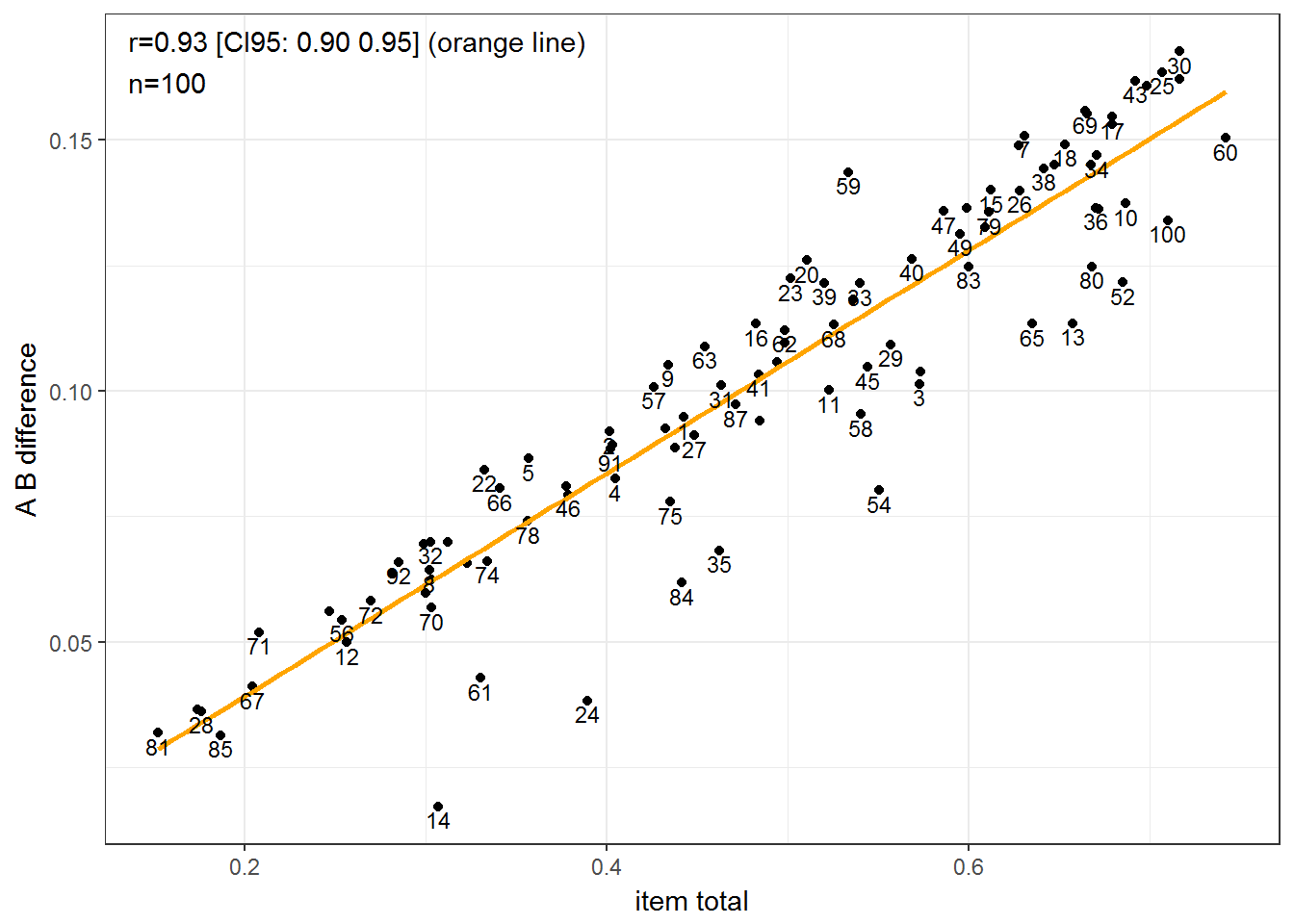GG_scatter(d2_items, "item_total", "A_C_difference")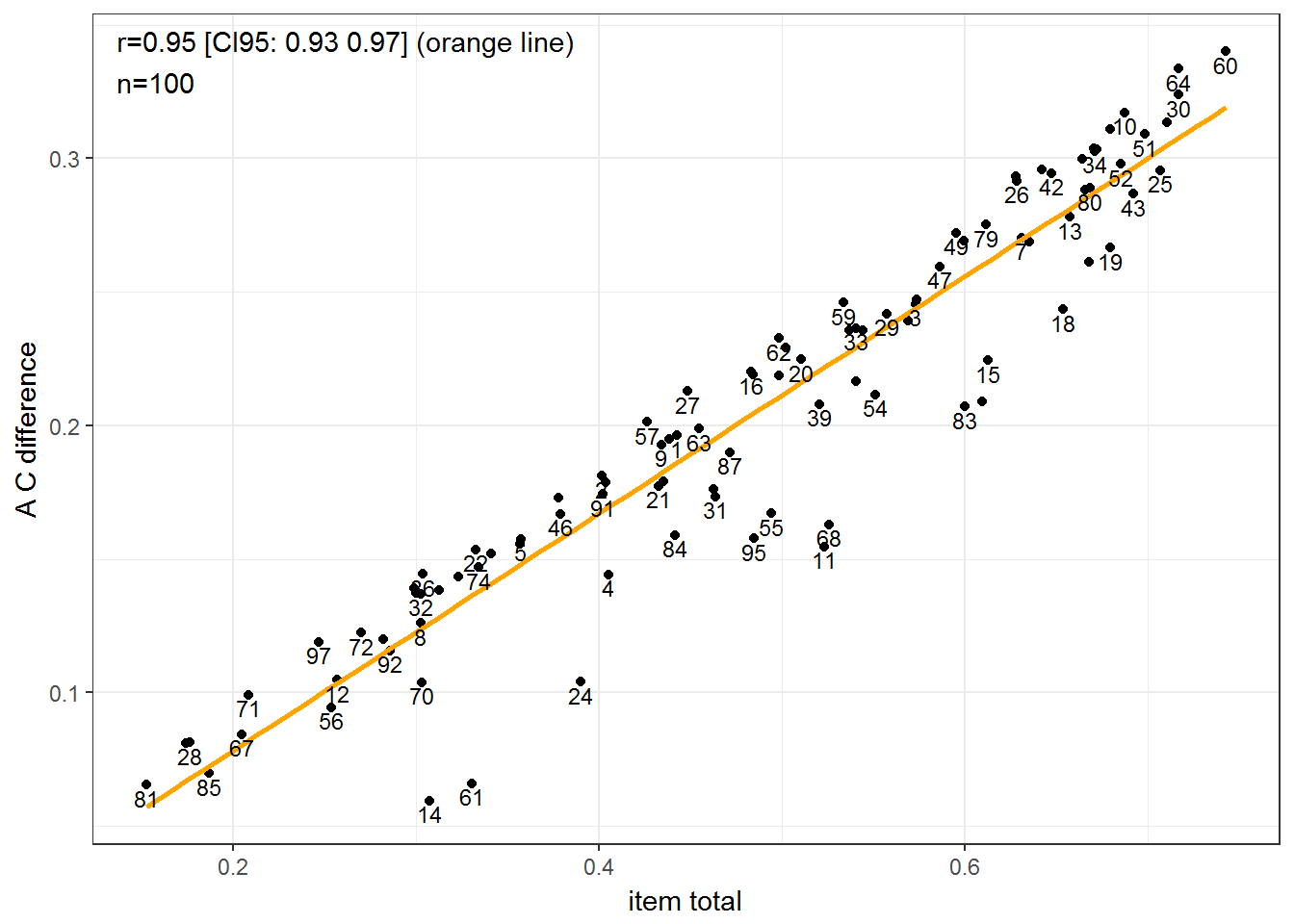GG_scatter(d2_items, "item_total", "B_C_difference")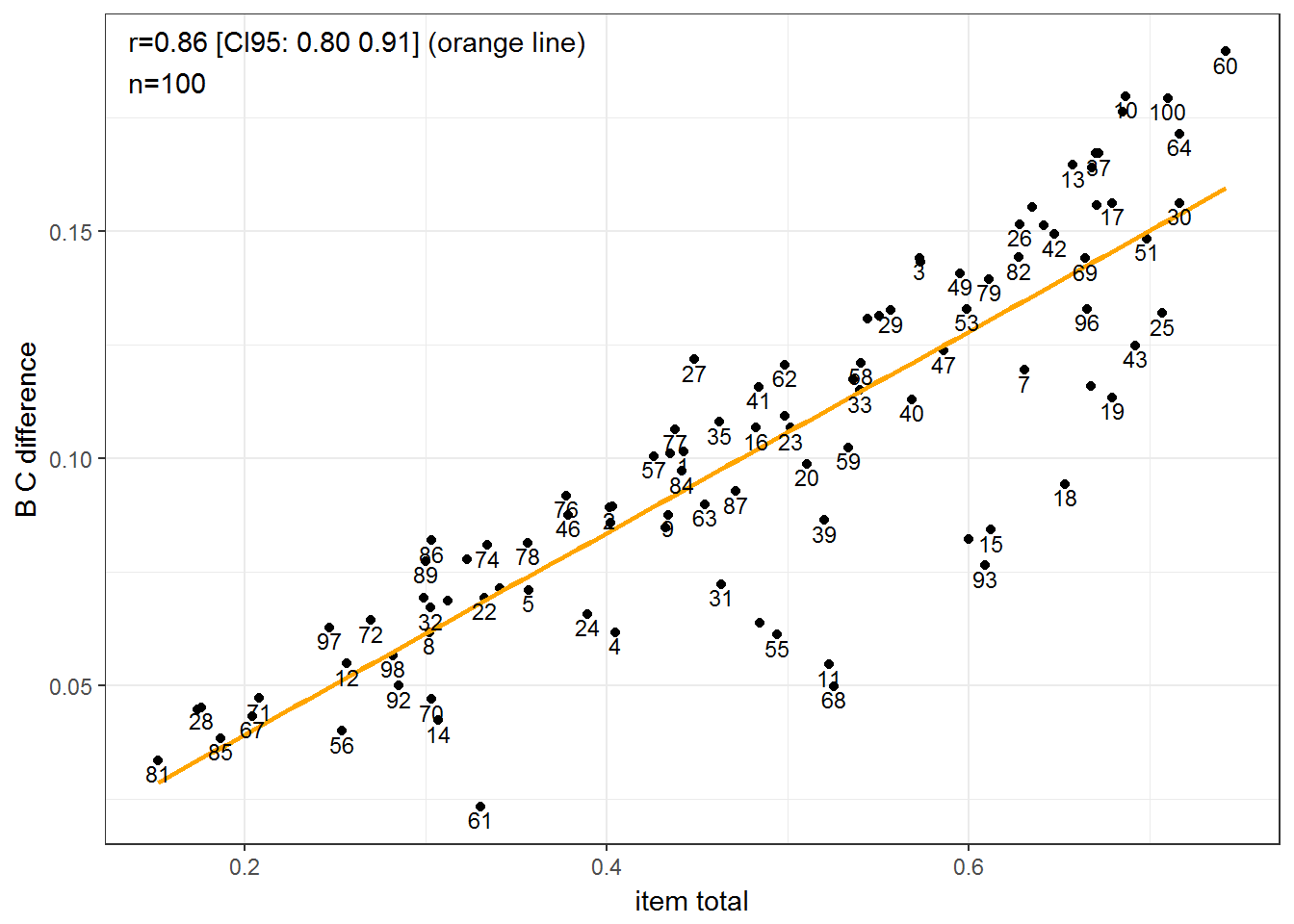We can see the problem. We know the score differences between groups are entirely due to g, but the correlations are not quite 1.00 and we see some odd outlying points. There seems to be something influencing the relationship. What if we instead used the latent (biserial) correlations? #calculate biserial item-total correlations # d2_items$item_total_latent = hetcor(d2_data[3:ncol(d2_data)], std.err = F)$correlations[-1, 1] quietly({d2_items$item_total_latent = map_dbl(df_subset_by_pattern(d2_data, pattern = "item_"), function(x) biserial(d2_data$total, x))}) ## function (...) ## capture_output(.f(...)) ## <environment: 0x00000000147128e8> #plots GG_scatter(d2_items, "item_total_latent", "A_B_difference")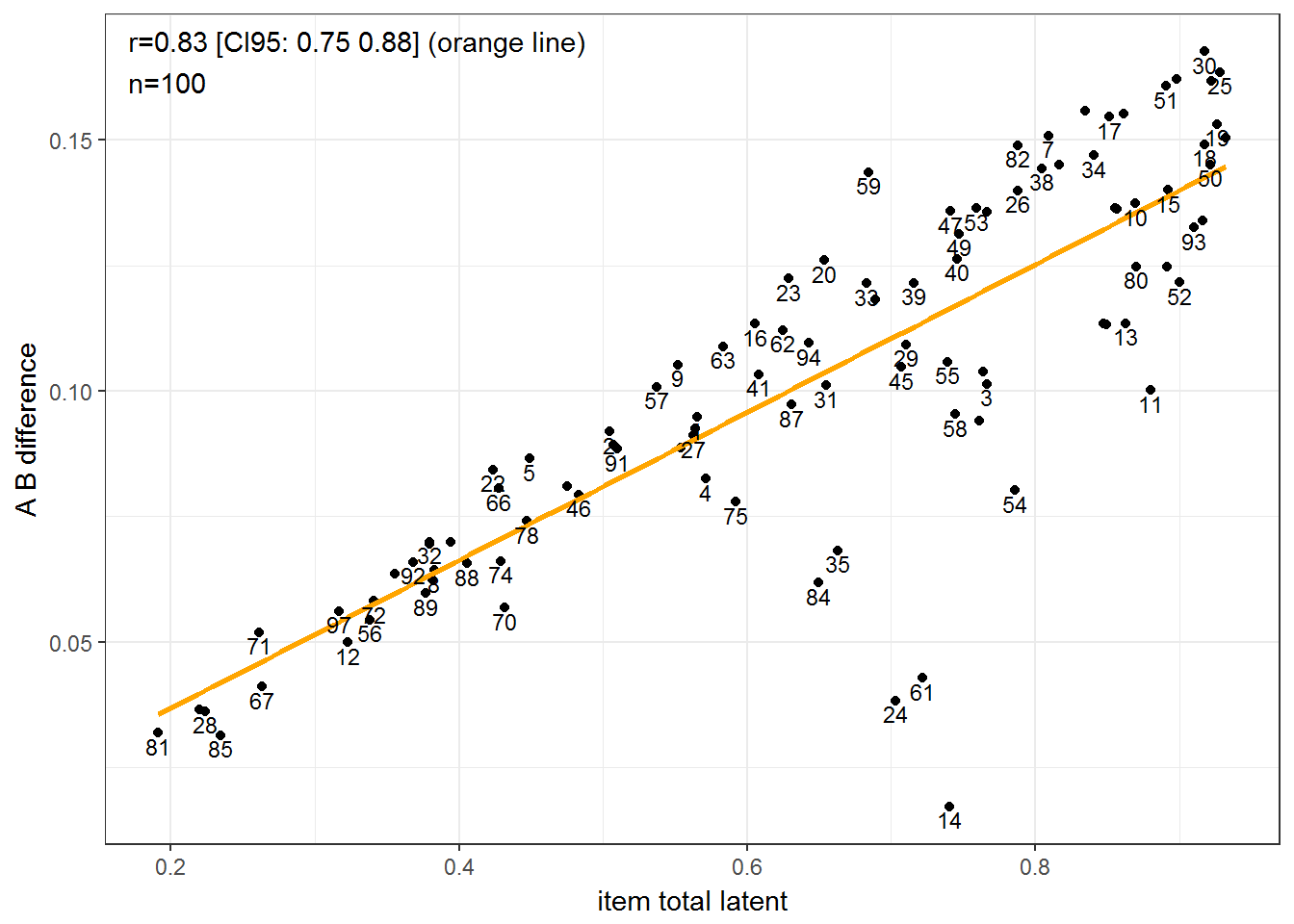GG_scatter(d2_items, "item_total_latent", "A_C_difference")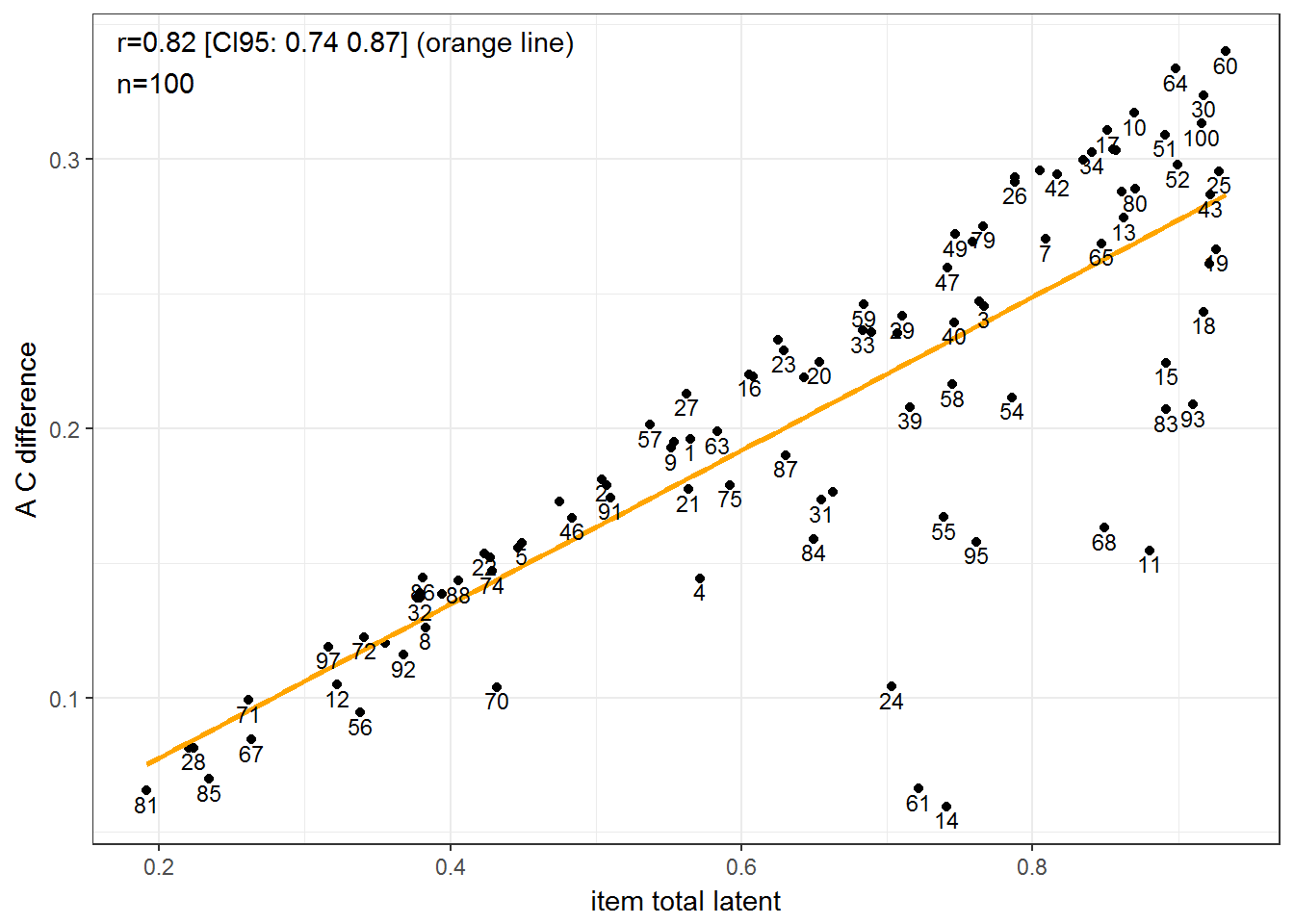GG_scatter(d2_items, "item_total_latent", "B_C_difference")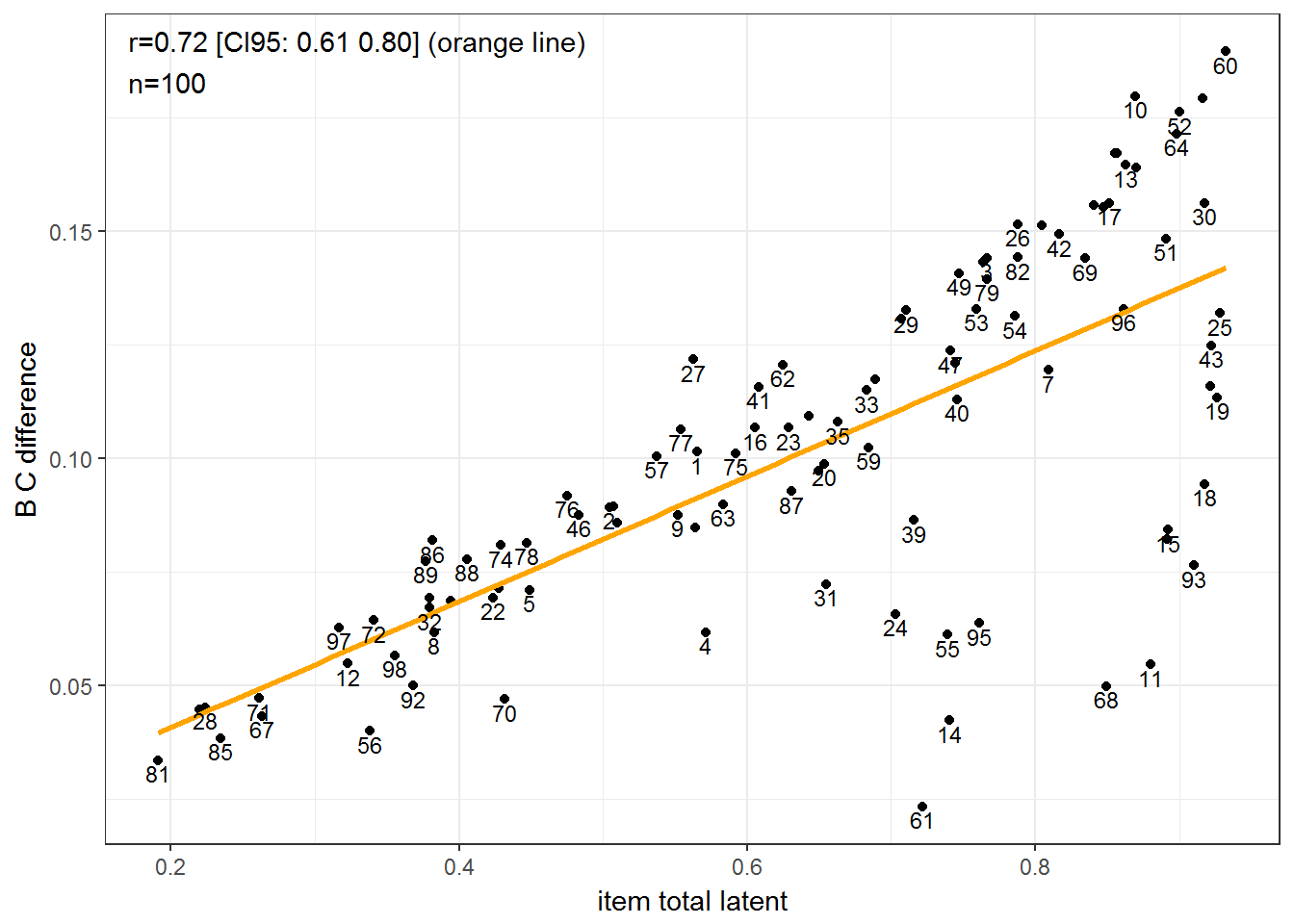Surprisingly, perhaps, these correlations are even odder than before. There’s clearly something else amiss. # Item response theory? What if we take the plunge and use item response theory factor analysis? Note that doing this analysis requires case-level data or at least a correlation matrix of latent correlations between items. This approach seriously limuts our ability to use older datasets due to lack of data sharing. How do the loadings from this analysis relate to the latent item-total correlations? #IRT fa irt_fa = irt.fa(d2_data[-(1:3)], plot = F, sort = F) d2_items$irt_loadings = irt_fa$fa$loadings %>% as.vector

#plot
GG_scatter(d2_items, "item_total_latent", "irt_loadings")